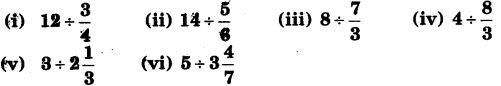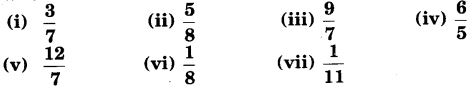# Class 7 Maths NCERT Solutions for Chapter 2 Fractions and Decimals EX – 2.4

## Fractions and Decimals

Question 1.
Find :Solution:

Question 2.
Find the reciprocal of each of the following fractions. Classify the reciprocals as proper fractions, improper fractions and whole numbers.Solution:

Question 3.
Find :Solution:

Question 4.
Find:Solution: